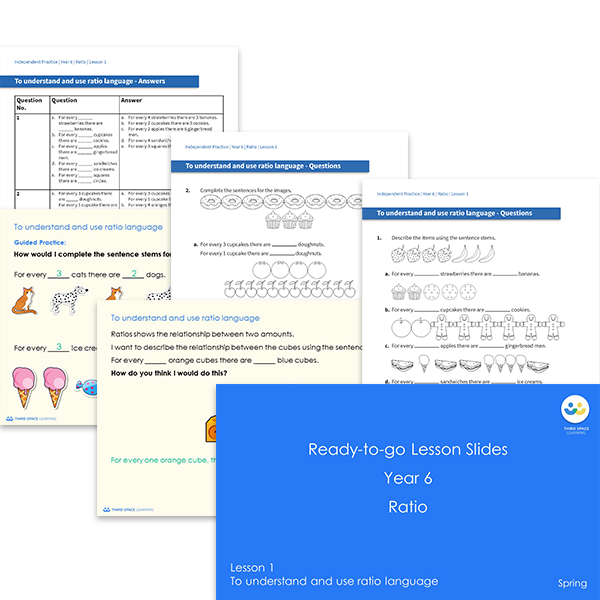# Ready-to-go lessons Year 6 Ratio (Spring Block 6) Slides and Worksheets

Ratio ready-to-go lessons for Year 6 includes 7 PowerPoints with matching worksheets ready for you to use with a class. These resources follow a mastery approach to mathematics teaching, similar to schemes such as White Rose Maths and Mathematics Mastery. The PowerPoints are fully editable with detailed notes for every teaching slide. Each associated worksheet is divided into three parts with a range of activities to complete across the block.

This Year 6 Ratio pack contains 7 fully editable PowerPoints and 7 worksheets.

The slides and worksheets cover:

• Using ratio language
• Understanding the relationship between fractions and ratio
• Introducing the ratio symbol
• Calculating ratio
• Using and calculating ratio factors
• Solving ratio and proportion problems

Year 6

Ratio and Proportion